# MCAT Physical : Thermodynamics of Phase Changes

## Example Questions

← Previous 1

### Example Question #1 : Thermodynamics Of Phase Changes

A scientist prepares an experiment to demonstrate the second law of thermodynamics for a chemistry class. In order to conduct the experiment, the scientist brings the class outside in January and gathers a cup of water and a portable stove.

The temperature outside is –10 degrees Celsius. The scientist asks the students to consider the following when answering his questions:

Gibbs Free Energy Formula:

ΔG = ΔH – TΔS

Liquid-Solid Water Phase Change Reaction:

H2O(l) ⇌ H2O(s) + X

The scientist prepares two scenarios.

Scenario 1:

The scientist buries the cup of water outside in the snow, returns to the classroom with his class for one hour, and the class then checks on the cup. They find that the water has frozen in the cup.

Scenario 2:

The scientist then places the frozen cup of water on the stove and starts the gas. The class finds that the water melts quickly.

After the water melts, the scientist asks the students to consider two hypothetical scenarios as a thought experiment.

Scenario 3:

Once the liquid water at the end of scenario 2 melts completely, the scientist turns off the gas and monitors what happens to the water. Despite being in the cold air, the water never freezes.

Scenario 4:

The scientist takes the frozen water from the end of scenario 1, puts it on the active stove, and the water remains frozen.

Which of the following are possible quantities for the “X” present on the right side of the Liquid-Solid Water Phase Change Reaction?

I. Entropy

II. Heat

III. Water Vapor

I only

I and III only

I and II

II only

I, II, and III

II only

Explanation:

As water freezes, it releases heat into the universe to balance the local loss of entropy encountered by ordering atoms into a rigid solid structure. Entropy, in contrast, is not a commodity that can be released by a reaction in the same way as heat. Also, water vapor would not be released on only one side of the equation. Water vapor would exist with in equilibrium with both solid and liquid, but would not appear as an additional product with the solid phase in this reaction.

### Example Question #2 : Thermodynamics Of Phase Changes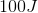of energy is added to a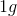sample, which undergoes a phase change from liquid to gas as a result. The temperature remains constant throughout the process. What does thequantity represent?

Specific heat of vaporization

Heat of vaporization

Specific heat capacity

Enthalpy of vaporization

Specific heat of vaporization

Explanation:

The energy required to cause a liquid-gas phase change is called the heat of vaporization, and is usually given in the units of kilojoules per mole. Enthalpy of vaporization and heat of vaporization are the same quantity.

When the heat of vaporization is given for a single gram of substance, with the units of Joules per gram, it is termed the specific heat of vaporization. This is the quantity given in the question: the energy required to vaporize exactly one gram of the sample.

Specific heat capacity refers to the energy needed to raise the temperature of one gram of a sample by one degree Celsius.

### Example Question #3 : Thermodynamics Of Phase Changes

The following diagram shows the temperature of a substance as constant heat is supplied. Suppose the substance began in a solid state.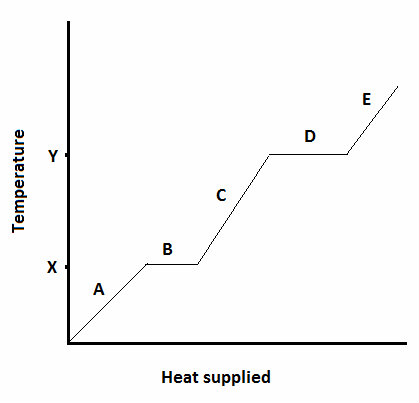What is the freezing point of the substance?

B

X

D

Y

X

Explanation:

Freezing point is always a temperature, meaning it must correspond to a single point on the y-axis. During the freezing/melting process, heat is absorbed/released from the system without any change in temperature. This results in the temperature plateau at the freezing point.

While the substance is freezing during interval B, the freezing point is the temperature at which it freezes, X.

### Example Question #4 : Thermodynamics Of Phase Changes

The following diagram shows the temperature of a substance as constant heat is supplied.  Suppose the substance began in a solid state.The slope of the graph is zero at intervals B and D because __________.

no energy is being supplied to the system

the heat that is supplied is being used to overcome intermolecular forces

the substance is undergoing fusion at these intervals

no phase changes are taking place at these intervals

the heat that is supplied is being used to overcome intermolecular forces

Explanation:

During intervals B and D, fusion and vaporization are taking place, respectively. Instead of raising the temperature of the substance, the energy that is added during these phase changes is used to overcome intermolecular forces. The energy breaks the attraction between particles, allowing them to separate and gain the properties of a higher energy phase (liquid or gas).

When the slope is not zero, the phase is steady and the added heat energy is used to increase the molecular kinetic energy of the particles, resulting in a temperature increase.

### Example Question #5 : Thermodynamics Of Phase Changes

The molar heats of fusion and vaporization for water are given below: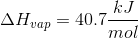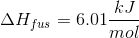A certain amount of snow is formed in the atmosphere, and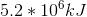of energy is released. How much water was solidified?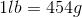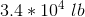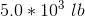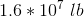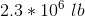Explanation:

Use the given molar heat of fusion to calculate the amount of snow formed. Remember that heats of fusion and solidification are opposite processes, so the magnitudes of molar heats of fusion and solidification are the same and signs are opposite. This indicates that fusion (melting) is endothermic, while solidification is exothermic.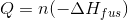The given change in energy will be negative, since the question states that it is released from the system into the atmosphere.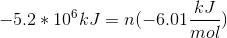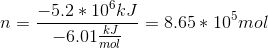Convert moles to grams, and grams to pounds.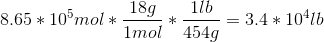### Example Question #6 : Thermodynamics Of Phase Changes

The molar heats of fusion and vaporization for water are given below: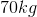of water solidify and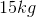of water vaporize within a closed system. What is the change in energy of the surroundings?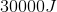of energy is absorbed from the surroundings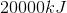of energy is released to the surroundings

The system experiences no overall change in energy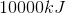of energy is absorbed from the surroundingsof energy is absorbed from the surroundings

Explanation:

We must use the given heats of fusion and vaporization to calculate the energy change involved in these two processes.

First, calculate the energy change whenof water solidifies. Convert the mass to moles and multiply by the heat released during freezing. This value will be equal in magnitude, but opposite in sign to the heat of fusion.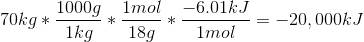From this calculation we find that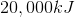of heat is released into the surroundings (a negative sign denotes an exothermic process).

Next, find the energy change associated with the vaporization ofof water, using the given heat of vaporization: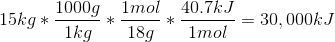We find that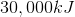of energy is absorbed when this quantity of water is vaporized.

Adding the two together we find a total of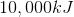.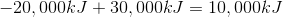Since this is a positive number, that means that the energy is absorbed from the surroundings (endothermic).

### Example Question #7 : Thermodynamics Of Phase Changes

The enthalpy of fusion for water is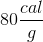.

The specific heat capacity for ice is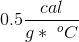.

The specific heat capacity for water is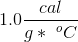.

How much heat is necessary to raise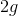of water from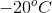to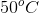?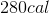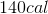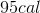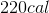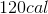Explanation:

Solving this problem means solving for three steps.

1. The heat needed to raise the temperature from –20oC to 0oC.

2. Melting the ice (changing phases).

3. Raising the water temperature from 0oC to 50oC.

Steps 1 and 3 are both solved by the equation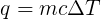.

Step 2 is solved using the enthalpy of fusion, and is multiplied by the number of grams being melted: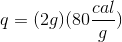.

Combining the steps, we get the following expression.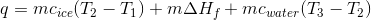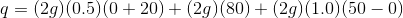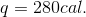### Example Question #8 : Thermodynamics Of Phase Changes

The molar heats of fusion and vaporization for water are given below:Which of the following is not true?

Vaporization is an endothermic process

The heat of condensation is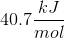Solidification is an exothermic process

It takes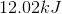to melt two moles of water

The heat of condensation isExplanation:

Vaporization and condensation refer to the transition from liquid to gas and from gas to liquid, respectively. Fusion and freezing, in contrast, refer to the transition from solid to liquid and from liquid to solid, respectively. Vaporization (boiling) and fusion (melting) each require an input of energy, making them endothermic processes with positive changes in enthalpy. Condensation and freezing result in a decrease in energy and an output of enthalpy, making them exothermic.

The given enthalpy of fusion tells us that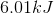are consumed for every mole of water. If two moles of water are present, thenare consumed during melting.

Enthalpy of vaporization will be equal and opposite the enthalpy of condensation. Since condensation is exothermic, heat will be released and the change in enthalpy must be negative (not positive).

Heat of condensation: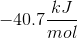### Example Question #9 : Thermodynamics Of Phase Changes

The molar heats of fusion and vaporization for water are given below:Given the following information, which substance would be expected to have an intermolecular force strength similar to that of water?

 Substance ΔHfus ΔHvap Acetone 5.72 kJ/mol 29.1 kJ/mol Ethanol 4.60 kJ/mol 43.5 kJ/mol Hydrogen 0.12 kJ/mol 0.90 kJ/mol Ammonia 5.65 kJ/mol 23.4 kJ/mol

Hydrogen

Ethanol and ammonia

Ethanol

Acetone and ammonia

Ethanol

Explanation:

Intermolecular forces play a key role in determining the energy required for phase changes. Strong intermolecular forces result in more resistance to changes that result in greater distance between molecules (greater entropy), as the forces cause the molecules to "stick" to one another. When a liquid is vaporized, the strength of the intermolecular force is overcome; similar heats of vaporization indicate similar intermolecular forces. A similar concept governs the transition from solid to liquid. If two compounds share similar enthalpies of fusion and vaporization, then they likely have similar intermolecular forces.

In the given table, ethanol enthalpy values are most similar to those of water, meaning it likely has similar intermolecular forces.

### Example Question #1151 : Mcat Physical Sciences

The following diagram shows the temperature of a substance as constant heat is supplied. Suppose the substance began in a solid state.If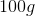of the substance begins at interval E and ends at interval C, the substance __________ and energy is __________.

solidifies . . . released

vaporizes . . . released

condenses . . . absorbed

condenses . . . released